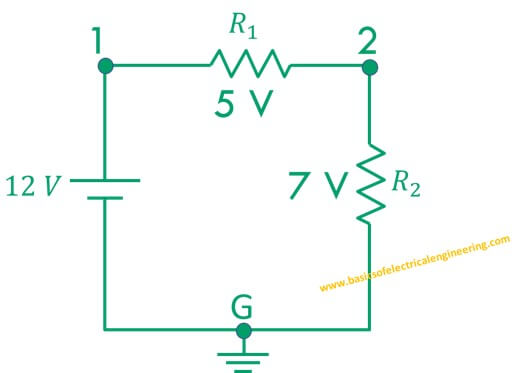# Basics of Electrical Engineering

Learn the basics of Electrical Engineering.

Ground, neutral and earth ground are three basic concepts of Electrical Engineering. In this post, you'll learn the difference between them.
Let's take a look at current flow in any circuit:
The electrical current in any circuit travels from the positive terminal of the battery passes through the load and then flows back to the negative terminal.

### What is ground

The electrical ground in any circuit is a common reference point. The ground node is considered at a potential difference of zero and all other readings are taken with the reference of the ground.

The figure below displays a voltage divider circuit with two resistors.The point G represents reference point with potential 0. Whereas point 1 represents reference point 1. Now if we say that potential difference at point 1 is 12 V. We actually mean that with reference to point G the potential at 1 is 12 V. Similarly at point 2 the potential of 7 V means we are comparing that point with the reference G.

### What is neutral

The neutral is a return conductor which carries current back to the source. In the previous circuit, the wire which connects 7 V resistor to the negative terminal of the battery is neutral.
The neutral in the previous circuit is highlighted with black colored wire for sake of understanding.

### What is earth ground

The earth ground refers to a rod which is inserted into the earth soil.

The metallic body of the appliance is usually connected to the earth ground for providing protection.

The video below clearly illustrates it: